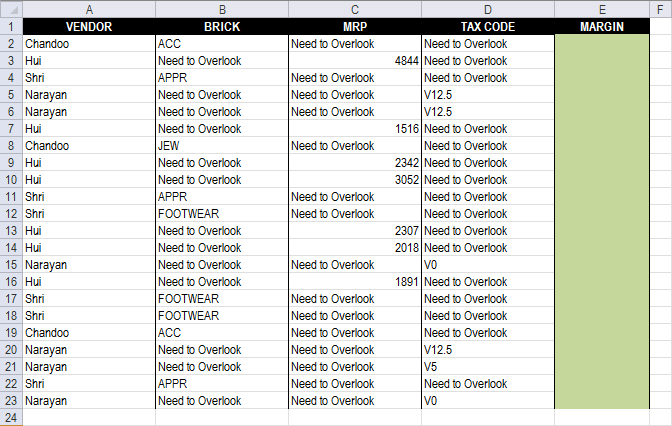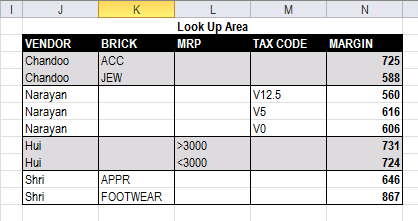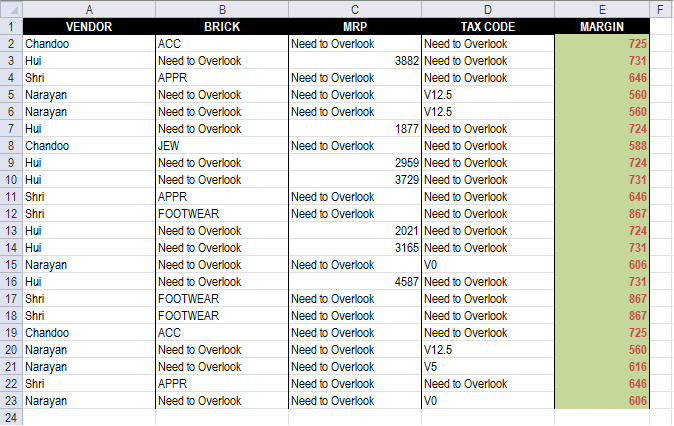Author: Oscar Cronquist Article last updated on March 01, 2013

Debraj Roy asks:

Hi Oscar,

I failed to search for "Ask" Section.. so submitting my query here.. Apologize for hijacking someones post,, and also for cross-post..

http://chandoo.org/forums/topic/lookup-using-multiple-condition

Can You please help me to create a drag-able FORMULA to get Margin.. via multiple Condition..

* Each Vendor has only one type of MARGIN decider (MRP or BRICK or TAX Code)
* According to Margin decider, I need to get MARGIN for the VENDOR..

i.e Vendor 1 (Chandoo) 's Margin Decider is BRICK..
If brick is ACC then Margin is 548, if Brick is JEW then Margin is 786.. (Check Table2 for lookupArea and Margin Decider..LookUpArea..* suggestion for changing Design of LookUpArea's is appreciable.
* use of HELPER column is also acceptable..

https://dl.dropbox.com/u/78831150/Excel/MultipleLookup.xlsx

You can download the VBA version for your reference..
https://dl.dropbox.com/u/78831150/Excel/MultipleLookup.xlsm

Regards,
Deb

### Answer:Array formula in cell E2:

=INDEX(\$N\$3:\$N\$11, MATCH(1, COUNTIFS(INDEX((B2, C2, D2), , , MATCH(TRUE, B2:D2<>"Need to Overlook", 0)), INDEX((\$K\$3:\$K\$11, \$L\$3:\$L\$11, \$M\$3:\$M\$11), , , MATCH(TRUE, B2:D2<>"Need to Overlook", 0)), A2, \$J\$3:\$J\$11), 0))

How to enter an array formula

1. Select cell E2
2. Paste array formula to formula bar
3. Press and hold CTRL + SHIFT
4. Press Enter

How to copy array formula

1. Select cell E2
2. Copy cell E2 (Ctrl + c)
3. Select cell range E3:E23
4. Paste (Ctrl + v)

### Explaining array formula in cell E2

Step 1 - Determine which cell range to use

MATCH(TRUE, B2:D2<>"Need to Overlook", 0)

becomes

MATCH(TRUE, {"ACC","Need to Overlook","Need to Overlook"}<>"Need to Overlook", 0)

becomes

MATCH(TRUE, {TRUE,FALSE,FALSE}, 0)

and returns 1.

Step 2 - Return cell reference

INDEX((B2, C2, D2), , , MATCH(TRUE, B2:D2<>"Need to Overlook", 0))

becomes

INDEX((B2, C2, D2), , , 1)

and returns cell reference B2

Step 3 - Find matching row

COUNTIFS(INDEX((B2, C2, D2), , , MATCH(TRUE, B2:D2<>"Need to Overlook", 0)), INDEX((\$K\$3:\$K\$11, \$L\$3:\$L\$11, \$M\$3:\$M\$11), , , MATCH(TRUE, B2:D2<>"Need to Overlook", 0)), A2, \$J\$3:\$J\$11)

becomes

COUNTIFS(B2, \$K\$3:\$K\$11, A2, \$J\$3:\$J\$11)

and returns {1;0;0;0;0;0;0;0;0}

Step 4 - Calculate relative position of row

MATCH(1, COUNTIFS(INDEX((B2, C2, D2), , , MATCH(TRUE, B2:D2<>"Need to Overlook", 0)), INDEX((\$K\$3:\$K\$11, \$L\$3:\$L\$11, \$M\$3:\$M\$11), , , MATCH(TRUE, B2:D2<>"Need to Overlook", 0)), A2, \$J\$3:\$J\$11), 0)

becomes

MATCH(1, {1;0;0;0;0;0;0;0;0}, 0)

and returns 1.

Step 5 - Return margin value

=INDEX(\$N\$3:\$N\$11, MATCH(1, COUNTIFS(INDEX((B2, C2, D2), , , MATCH(TRUE, B2:D2<>"Need to Overlook", 0)), INDEX((\$K\$3:\$K\$11, \$L\$3:\$L\$11, \$M\$3:\$M\$11), , , MATCH(TRUE, B2:D2<>"Need to Overlook", 0)), A2, \$J\$3:\$J\$11), 0))

becomes

=INDEX(\$N\$3:\$N\$11, 1)

becomes

=INDEX({725; 588; 560; 616; 606; 731; 724; 646; 867}, 1)

and returns 725 in cell E2.

### Download excel *.xlsx file

MultipleLookup_solution1.xlsx